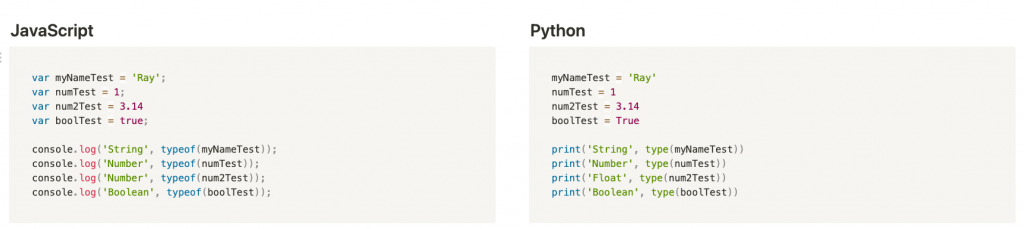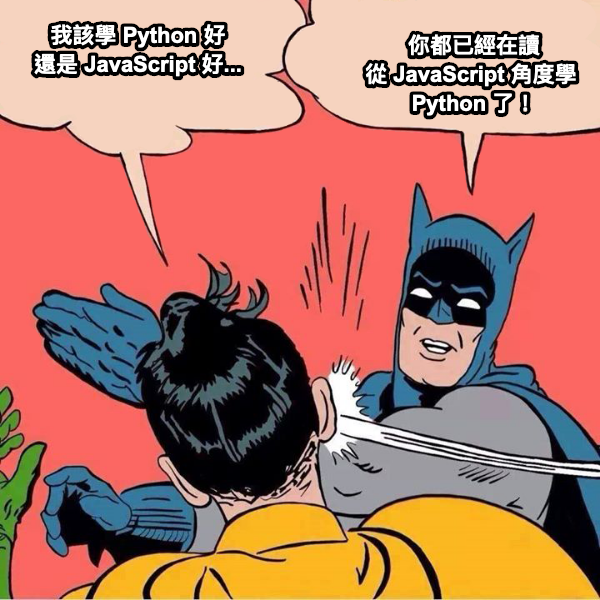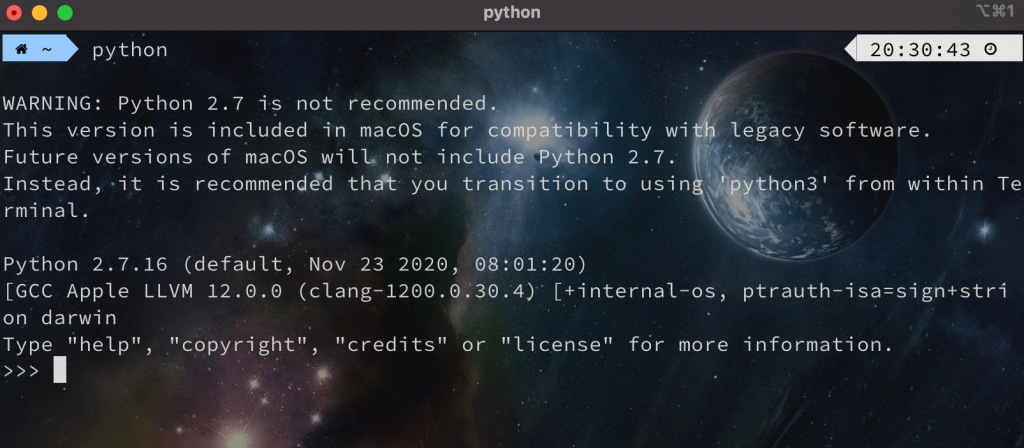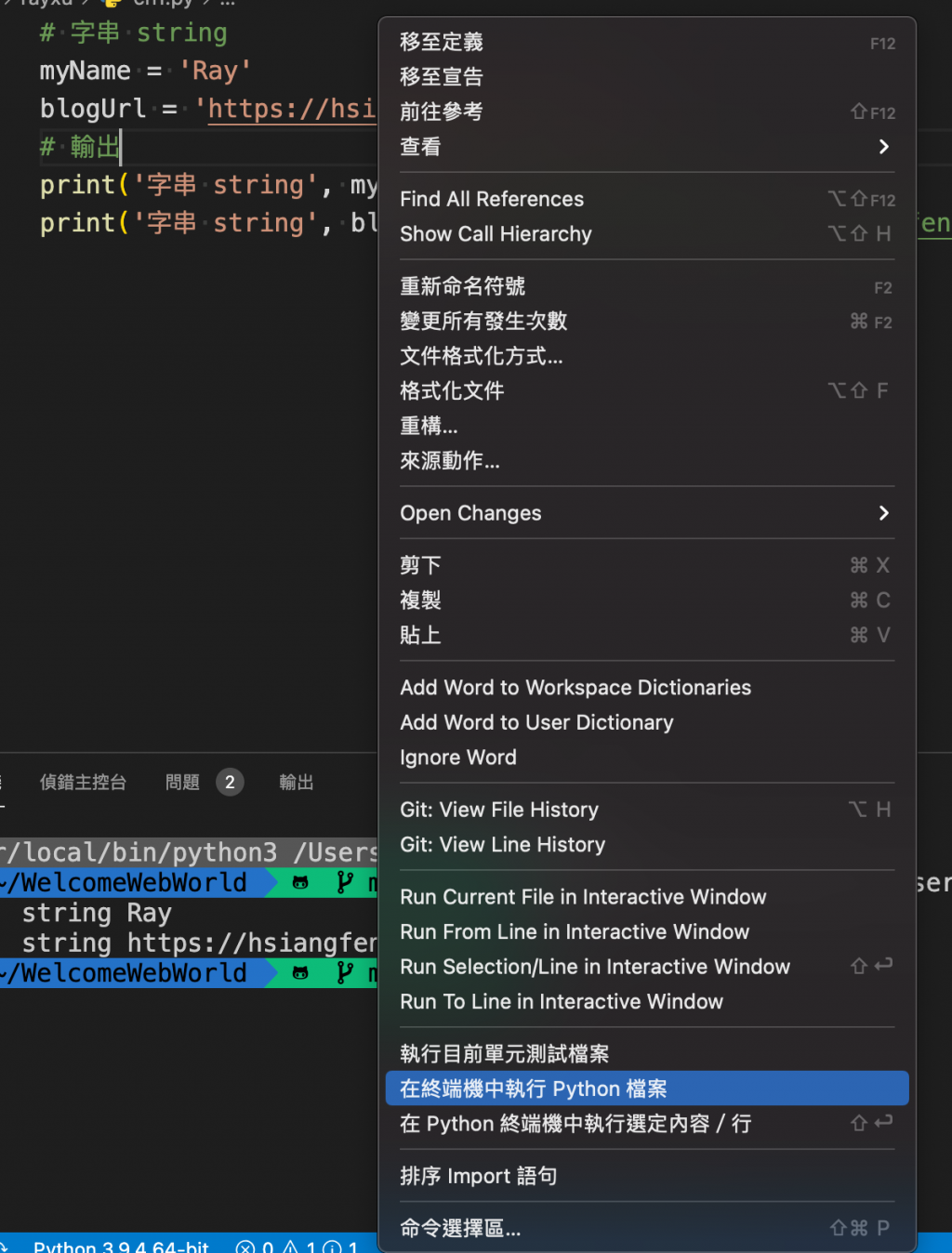DAY 4
1
Software Development

前言

oh！終於要開始學習寫 Python 了呢！(被揍)，都混了三天，我如果再不開始寫 Python 的話，我想應該一推人準備退訂閱了，但是在開始學習之前，我們會先從最基礎的型別開始學習，那麼這邊也會盡可能使用 JavaScript 兩者語法上的差異去做比較與學習唷。

型別• String - 字串
• '這是一個字串'
• "這也是一個字串"
• Boolean - 布林
• true
• false
• Number - 數字
• 100000
• 3.141592653
• Undefined - 未定義
• Null - 空值

• Bigint - 整數數值
• 9007199254740991n
• Symbol - Symbol

• 數值型別
• 字串型別
• 容器型別

• 數值型別
• int - 整數
• floats - 浮點數
• boolean - 布林
• 字串型別
• string - 就是字串你還想怎樣？(前面開玩笑的。)

var num = 1.23456;
var num2 = 123;
console.log('Number', typeof(num)); // Number number
console.log('Number', typeof(num2)); // Number number

Undefined 就不說了，畢竟這是 JavaScript 的特殊型別，只要你有在變數宣告之前呼叫過變數的話，一定很常看到 Undefined (這部分又牽扯到提升就不提了。)：

console.log(myName); // undefined
var myName = 'Ray';

(注意，Python 不用加上結尾分號)

hello = Null # name 'Null' is not defined

hello = None
type(hello) # <class 'NoneType'>

(type 的語法與 JavaScript 的 typeof 相同，主要是查看型別用。)

變數

var myNameTest = 'Ray';
var numTest = 1;
var num2Test = 3.14
var boolTest = true;

console.log('String', typeof(myNameTest)); // String string
console.log('Number', typeof(numTest)); // Number number
console.log('Number', typeof(num2Test)); // Number number
console.log('Boolean', typeof(boolTest)); // Boolean boolean

Python 在宣告變數上與 JavaScript 是有一點點的不同，在 Python 中我們可以不使用任何的變數宣告關鍵字 (varlet or const) 來宣告變數，只需要直接撰寫變數名稱並賦予值或結果就可以了。

myNameTest = 'Ray'
numTest = 1
num2Test = 3.14
boolTest = True

boolTest = True
boolTest = False

myNameTest = 'Ray'
numTest = 1
num2Test = 3.14
boolTest = True

print('String', type(myNameTest))
print('Number', type(numTest))
print('Float', type(num2Test))
print('Boolean', type(boolTest))

(題外話一下 console.xxx 還有很多種，例如：console.dirconsole.table 等等，如果你不熟悉的話，可以閱讀我這一篇筆記 「淺談 JavaScript 中的 Debug 神器 Console」 了解一下。)重新賦值

var myName = 'Ray';
myName = 'Ray'; // 可以被重新賦值
let sayHi = 'Hello Ray';
sayHi = 'Ray Hello'; // 可以被重新賦值
const isRay = 'not Array';
isRay = 'is Array'; // 無法被重新賦值

var 與 ES6 語法又有些許不同，因此這邊不著墨在 JavaScript 宣告變數上，若想再深入了解可以閱讀我先前寫「淺談 var 與 let 的差異以及有無宣告變數的差異」這一篇。

let myName;
let myName; // Uncaught SyntaxError: redeclaration of let myNamemyNameTest = 'Ray'
print(myNameTest) # 'Ray'
myNameTest = 123
print(myNameTest) # 123

print(myNameTest) # NameError: name 'myNameTest' is not defined
myNameTest = 'Ray'
print(myNameTest)
myNameTest = 123
print(myNameTest)執行 Python(上圖出現 Python 2.7 可忽略)

• Window
• CTRL + D
• Mac
• Control + D

# 字串 string
myName = 'Ray'
blogUrl = 'https://hsiangfeng.github.io/'
# 輸出
print('字串 string', myName) # 字串 string Ray
print('字串 string', blogUrl) # 字串 string https://hsiangfeng.github.io/關於兔兔們